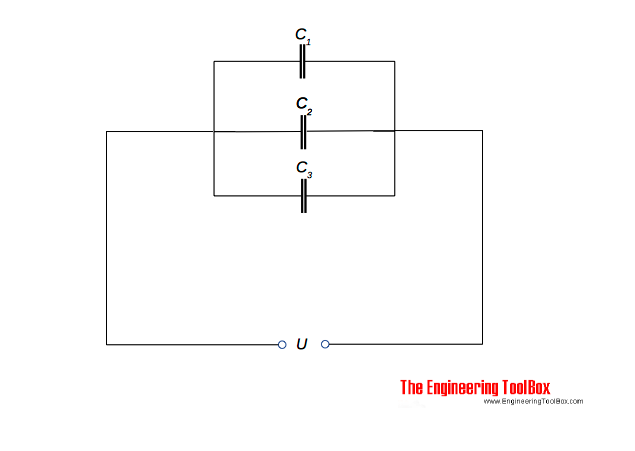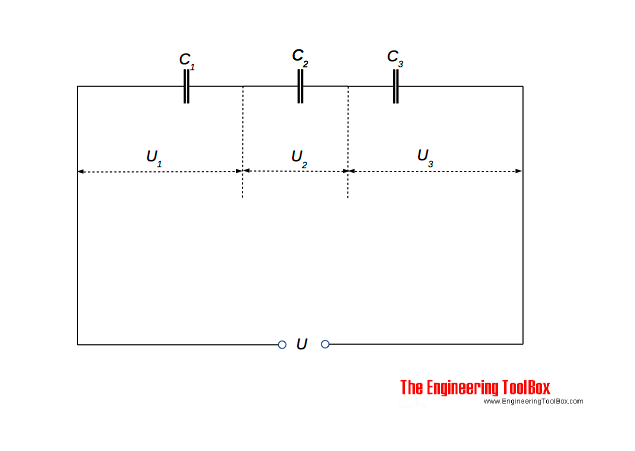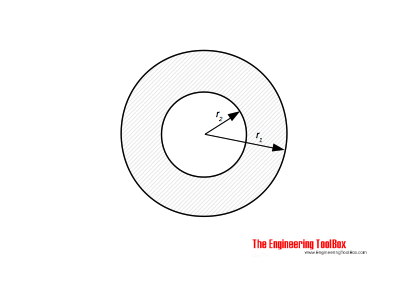Engineering ToolBox - Resources, Tools and Basic Information for Engineering and Design of Technical Applications!

# Capacitors - Parallel and Serial Connections

## Parallel and serial connected capacitor circuits.

### Capacitors in Parallel

Capacitors can be connected in parallel:The equivalent capacitance for parallel-connected capacitors can be calculated as

C = C1 + C2 + . . + Cn                     (1)

where

C = equivalent capacitance for the parallel connected circuit  (Farad, F, μF)

C1..n = capacitance capacitors (Farad, F, μF)

It is common to use µF as the unit for capacitance.

### Capacitors in Series

Capacitors can be connected in series:The equivalent capacitance for series-connected capacitors can be calculated as

1 / C = 1 / C1 + 1 / C2 + . . + 1 / Cn                  (2)

For the special case with two capacitors in series - the capacitance can be expressed as

1 / C = (C1 + C2) / (C1 C2)                     (2b)

- or transformed to

C = C1 C2 / (C1 + C2)                     (2c)

### Example - Capacitors Connected in Parallel and in Series

The equivalent capacitance of two capacitors with capacitance 10 μF and 20 μF  can be calculated as

in parallel

C = (10 μF) + (20 μF)

= 30 (μF)

in series

1 / C = 1 / (10 μF) + 1 / (20 μF)

= 0.15 (1/μF)

or

C = 1 / 0.15 (1/μF)

= 6.7 (μF)

### Capacitors in Series

Three capacitors C1 = 3 μF , C2 = 6 μF and C3 = 12 μF are connected in series as indicated in the figure above. The voltage supply to the circuit is 230 V.

The equivalent circuit capacitance can be calculated with (2)

1 / C = 1 / (3 μF) + 1 / (6 μF) + 1 / (12 μF)

= (4 + 2 + 1) / 12

= 0.58 1/μF

- or transformed

C = 12 / (4 + 2 + 1)

= 1.7 μF

The total charge in the circuit can be calculated with

Q = U C

where

Q = charge (coulomb, C)

U = electric potential (V)

- or with values

Q = (230 V) (1.7 10-6 F)

= 3.91 10-4 C

= 391 μC

Since the capacitors are connected in series - the charge is 391 μC on each of them.

The voltage across capacitor 1 can be calculated

U1 = Q / C1

= (391 μC) / (3 μF)

= 130 V

The voltage across capacitor 2 can be calculated

U2 = Q / C2

= (391 μC) / (6 μF)

= 65 V

The voltage across capacitor 3 can be calculated

U3 = Q / C3

= (391 μC) / (12 μF)

= 33 V

### Capacitance of Two Coaxial CylindersThe capacitance of two coaxial cylinders as indicated in the figure can be calculated as

C = 2 π εo εr l / ln(r2 / r1)                  (3)

where

εo = absolute permittivity, vacuum permittivity (8.85 10-12 F/m, Farad/m)

εr = relative permittivity

l = length of cylinders

r2 = radius of inner cylinder

r1 = radius of outer cylinder

## Related Topics

• ### Electrical

Electrical units, amps and electrical wiring, wire gauge and AWG, electrical formulas and motors.

## Related Documents

• ### ASME 31.3 Piping Materials - Allowable Pressure Calculator

Calculate ASME 31.3 allowable pressure
• ### Capacitors

Capacitors and capacitance - charge and unit of charge.
• ### Capacitors - Stored Energy

Potential power and energy stored in capacitors.
• ### Electrical Conductivity - Elements and other Materials

Electric conductance is the ability of a element to conduct an electric current.
• ### Electrical Inductance - Serial and Parallel Connections

Electrical inductance in serial and parallel connected inductors.
• ### Electrical Resistance in Serial and Parallel Networks

Resistors in parallel and serial connections.
• ### Relative Permittivity - the Dielectric Constant

Common materials and their relative permittivity.

## Engineering ToolBox - SketchUp Extension - Online 3D modeling!

Add standard and customized parametric components - like flange beams, lumbers, piping, stairs and more - to your Sketchup model with the Engineering ToolBox - SketchUp Extension - enabled for use with older versions of the amazing SketchUp Make and the newer "up to date" SketchUp Pro . Add the Engineering ToolBox extension to your SketchUp Make/Pro from the Extension Warehouse !

We don't collect information from our users. More about

## Citation

• The Engineering ToolBox (2008). Capacitors - Parallel and Serial Connections. [online] Available at: https://www.engineeringtoolbox.com/capacitors-parallel-series-d_1388.html [Accessed Day Month Year].

Modify the access date according your visit.

10.2.10